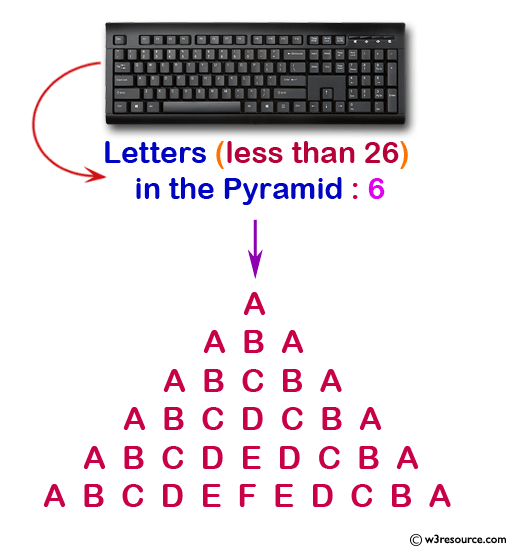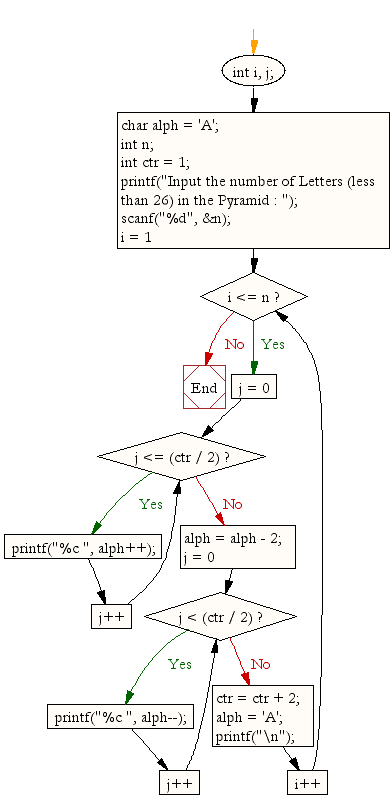﻿ C : Display the pattern like pyramid using the alphabet# C Exercises: Display the pattern like pyramid using the alphabet

## C For Loop: Exercise-40 with Solution

Write a C Program to display the pattern like pyramid using the alphabet. The pattern is as follows :

```     A
A B A
A B C B A
A B C D C B A
```

Pictorial Presentation:Sample Solution:

C Code:

``````#include <stdio.h>

void main()
{
int i, j;
char alph = 'A';
int n,blk;
int ctr = 1;

printf("Input the number of Letters (less than 26) in the Pyramid : ");
scanf("%d", &n);

for (i = 1; i <= n; i++)
{
for(blk=1;blk<=n-i;blk++)

printf("  ");
for (j = 0; j <= (ctr / 2); j++) {
printf("%c ", alph++);
}

alph = alph - 2;

for (j = 0; j < (ctr / 2); j++) {
printf("%c ", alph--);
}
ctr = ctr + 2;
alph = 'A';
printf("\n");
}
}
```
```

Sample Output:

```Input the number of Letters (less than 26) in the Pyramid : 6
A
A B A
A B C B A
A B C D C B A
A B C D E D C B A
A B C D E F E D C B A
```

Flowchart:C Programming Code Editor:

Improve this sample solution and post your code through Disqus.

What is the difficulty level of this exercise?

Test your Programming skills with w3resource's quiz.

﻿